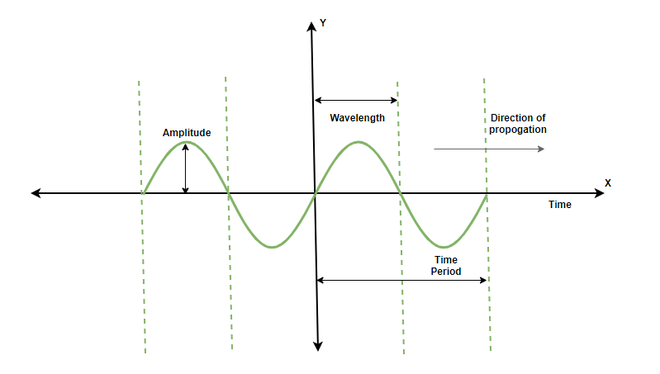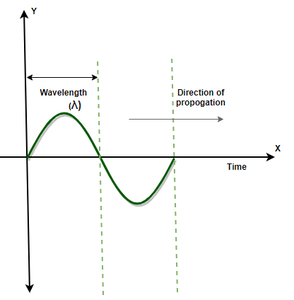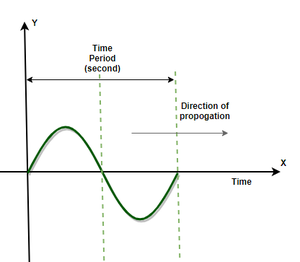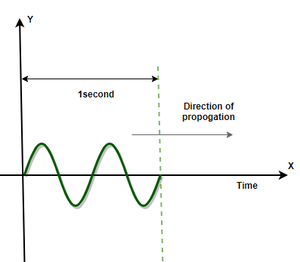# Properties of Waves

• Last Updated : 12 Oct, 2021

Waves are everywhere around us in real life, from the ripples that are created in water when throwing stones to the phones that are working around us. All these phenomena are happening because of waves. The waves are nothing but a disturbance in the medium they are traveling. It is essential to study their nature and properties to be able to predict their behavior in the places where their applications are needed. These properties also help us in predicting the resultant wave which originates when two or more waves in the medium meet. Let’s see these in detail.

### Waves

Wave is a disturbance in a medium that carries energy in them. For propagation, waves use elastic deformation, a variation of pressure or temperature, etc to propagate in the medium. It is not always necessary that all waves will require a medium for propagation, light waves can travel in a vacuum. Based on the way in which these waves travel in a medium, they can be classified into two types of waves – Longitudinal and Transverse waves.

### Properties of Waves

The characteristics of waves affect the waves and the propagation in different ways, there are 5 basic characteristics of a wave that are required to identify a wave. When the vibration is drawn in form of a wave, it becomes really easy to deduce the characteristics just by looking at the wave. The figure given below presents a birds-eye view of the characteristics of a transverse wave.Sound Wave Propagation

Wavelength (λ)

A Wavelength is defined as the minimum distance covered by the Wave before it starts repeating itself. The wavelength is actually a length parameter, hence, the SI unit of wavelength meters. The sound wave is longitude in nature, and the combination of the distance of one complete rarefaction and compression gives wavelength. The symbol for the wavelength used is λ.Time Period (T)

The Time period of a sound wave is defined as the time required for one complete cycle. One cycle of the wave is defined by one complete rarefaction and compression in the vibration. The time period is measured in seconds. The time period is also used to find out the frequency of the wave, the reciprocal of the time period gives the frequency of the wave.Frequency/Pitch (ν)

The frequency of the sound wave is defined as the number of cycles completed by the wave in 1 second. The frequency of the wave can also be defined as the number of vibrations created by the wave in one second. The SI unit of frequency is Hertz (Hz) and it is represented as the symbol nu (ν). Frequency is that parameter of the wave which does not change by changing the medium of the propagation. Hence, the frequency of the wave always remains the same.

An example of the frequency of the wave is,In the above figure, the frequency of the wave is to be determined. As it is clearly visible that two cycles are completed in 1 second. Hence, it can be concluded that the frequency of the wave is 2 Hz.

Formula to find out the frequency of the wave,

Frequency (ν) = 1/T,

Where T= Time Period

### Equation of a Wave

Notice that all these waves are in the form sinusoidal form. Thus, their equation also reflects the nature of a sinusoidal function. The function includes both the time “t” and the position “x”. For convenience let’s assume that the wave is transverse in nature. Denoting the displacement of the medium particles by “y”. The equation of the wave will be given by,

y = Asin(x, t)

⇒ y = Asin( x + vt)  or Asin(x – vt)

### Sample Problems

Question 1: The velocity of a wave is 600m/sec and the wavelength is given as 40Hz. What will be the wavelength of this wave?

The velocity of a wave is given as,

Velocity = frequency × wavelength

600= 40 × λ

λ =15meters

Hence, the wavelength of this wave is 15 meters.

Question 2: The Time period of a wave is 2 seconds and the wavelength is given as 20 meters. There is another wave with a time period of 6 seconds and a wavelength of 60 meters. Which of the two waves have more velocity.

Velocity of the first wave = 20/2

Velocity = 10m/sec

Velocity of the second wave= 60/6

Velocity = 10m/sec

Hence, the velocity of both the waves are equal.

Question 3: Find the amplitude and velocity of the wave whose equation is given below.

y = 3sin(x – 4t)

The equation of general wave is given by,

y = Asin( x + vt)  or Asin(x – vt)

Given:

y = 3sin(x – 4t)

Comparing these two equations,

A = 3 and v = 4

Question 4: Find the amplitude and velocity of the wave whose equation is given below.

y = 10sin(x – 5t)

The equation of general wave is given by,

y = Asin( x + vt)  or Asin(x – vt)

Given:

y = 10sin(x – 5t)

Comparing these two equations,

A = 10 and v = 5

Question 5: The Time period of a wave is given as 10 milliseconds. What will be the frequency of this wave?

As the formula known for the frequency of the wave is,

Frequency = 1/time period

Frequency = 1/(10 × 10-3)

Frequency = 1000/10

Frequency = 100Hz

Question 6: Two waves having Frequencies of 20Hz and 100Hz are given. Which wave has more Time period?

The Time period for the first wave = 1/frequency

= 1/20

= 0.05 second.

The Time period for the second wave= 1/frequency

= 1/100

= 0.01 second.

Hence, The time period of the first wave more than the Time period of the second wave.

My Personal Notes arrow_drop_up
Recommended Articles
Page :